# SQL Query to Convert VARCHAR to INT

• Difficulty Level : Basic
• Last Updated : 28 Oct, 2021

SQL Server’s CAST() and CONVERT() methods can be used to convert VARCHAR to INT. We’ll also look at the more efficient and secure approach to transform values from one data type to another.

### CAST()

The CAST() function in SQL Server is used to cast or transform a value or an expression from one data type to another.

Syntax :

`CAST ( expression AS target_type [ ( length ) ] )`

Parameters:

• expression – Any value of any type that will be converted.
• target_type – Target data type to which the value will be converted. e.g. INT, BIT, SQL_VARIANT, etc.
• length – Optional parameter that specifies the length of the target_type, default length is 30.

Let’s take an example where the CAST() function is used to convert VARCHAR to INT.

Query:

`SELECT CAST('1234' AS INT) AS Result;`

Output: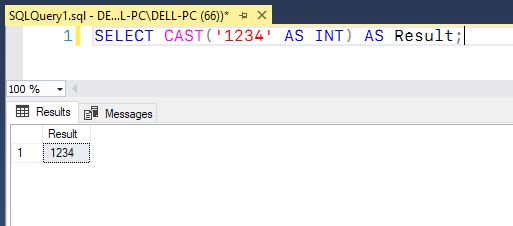### Convert()

In SQL Server, the CONVERT() function is used to convert a value of one type to another. Converting anything involves changing its shape or value.

Syntax :

`SELECT CONVERT ( target_type ( length ), expression )  `

Parameters:

• target_type – Data type to which the expression will be converted, e.g: INT, BIT, SQL_VARIANT, etc.
• length – It provides the length of the target_type. Length is not mandatory. The default length is set to 30.
• expression – expression is anything that will be converted.

In the below example, the CONVERT() function is used to convert VARCHAR to INT.

Query:

`SELECT CONVERT(INT,'5678') AS Result;`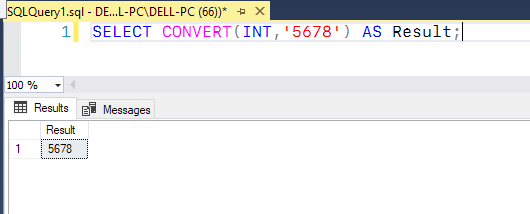Now Lets us discuss a more efficient approach to convert the values from one data type to another using SQL Server’s TRY_CAST() and  TRY_CONVERT() function:

### TRY_CAST()

The TRY_CAST() function attempts to cast the input value to a value of the given data type. If the cast is successful, it returns the value in the provided data; else, it returns NULL. However, if you request a conversion that is not valid, the TRY_CAST() method will return an error.

Syntax :

`TRY_CAST ( expression AS data_type [ ( length ) ] )  `

Parameters used:

• data_type: Valid data type into which the function will cast the expression.
• expression: Value to be cast.

Query:

`SELECT TRY_CAST('1234' as INT) as Result;`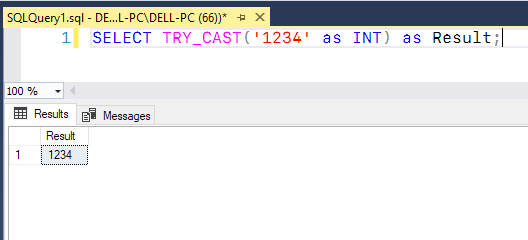Query:

`SELECT TRY_CAST('1234abc' as INT) as Result;`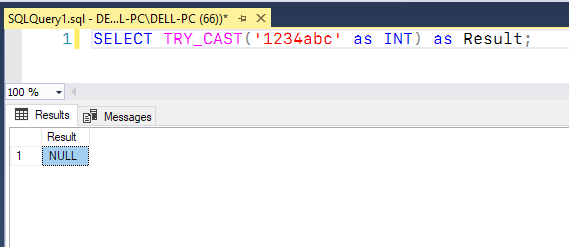### TRY_CONVERT()

The TRY_CONVERT() method attempts to convert the value supplied to it to the data type specified. If the cast is successful, it returns the value as the given data; else, it returns NULL. If you request a conversion that is explicitly forbidden, the TRY CONVERT() method will return an error.

Syntax :

`TRY_CONVERT ( data_type[(length)], expression [,style])`

Parameters used:

• data_type:  Valid data type into which the function will cast the expression.
• expression:  Value to be cast.
• style: Is a provided integer that specifies how the function will translate the expression.

Query:

`SELECT TRY_CONVERT( INT ,'5678') as Result;`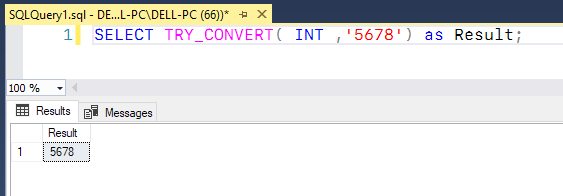Query:

`SELECT TRY_CONVERT( INT ,'56abc') as Result;`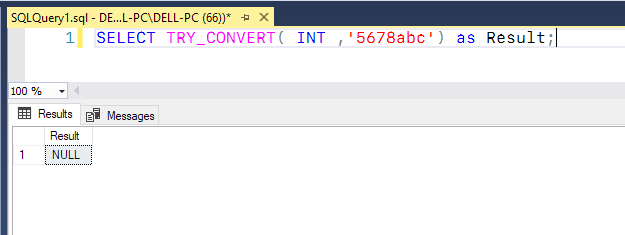My Personal Notes arrow_drop_up